Constructing a triangle when 3 sides known (SSS)

Practical Geometry
Concept wise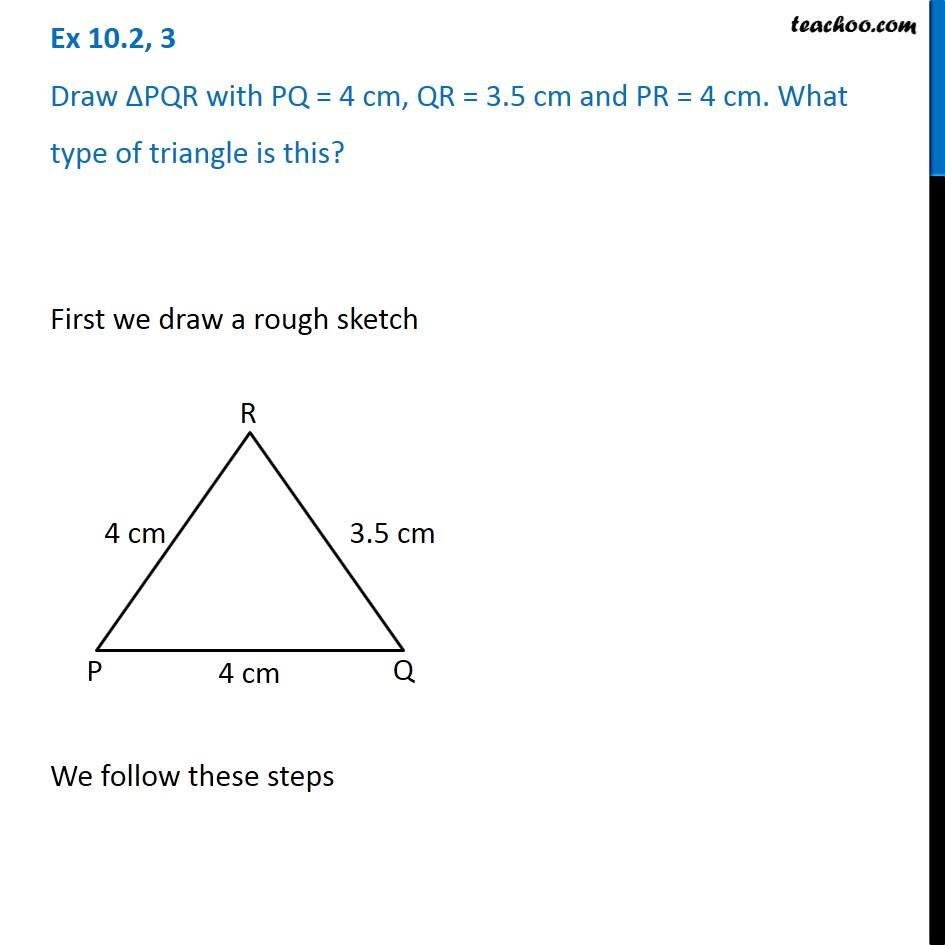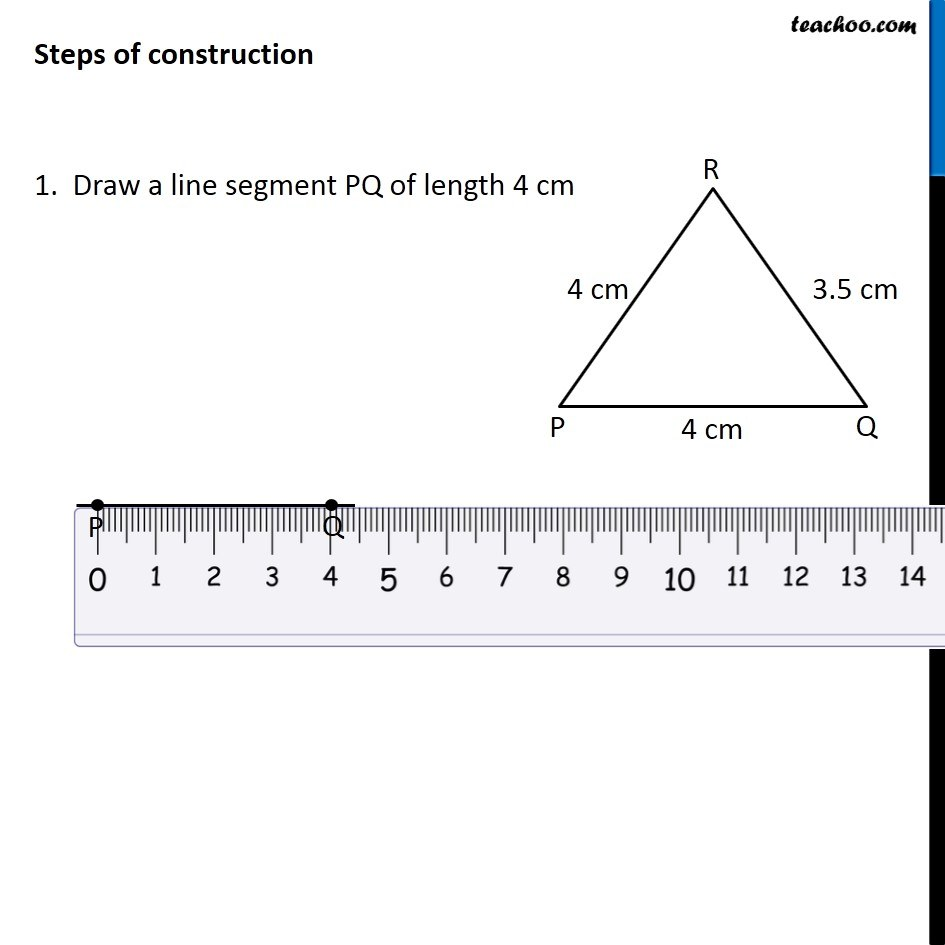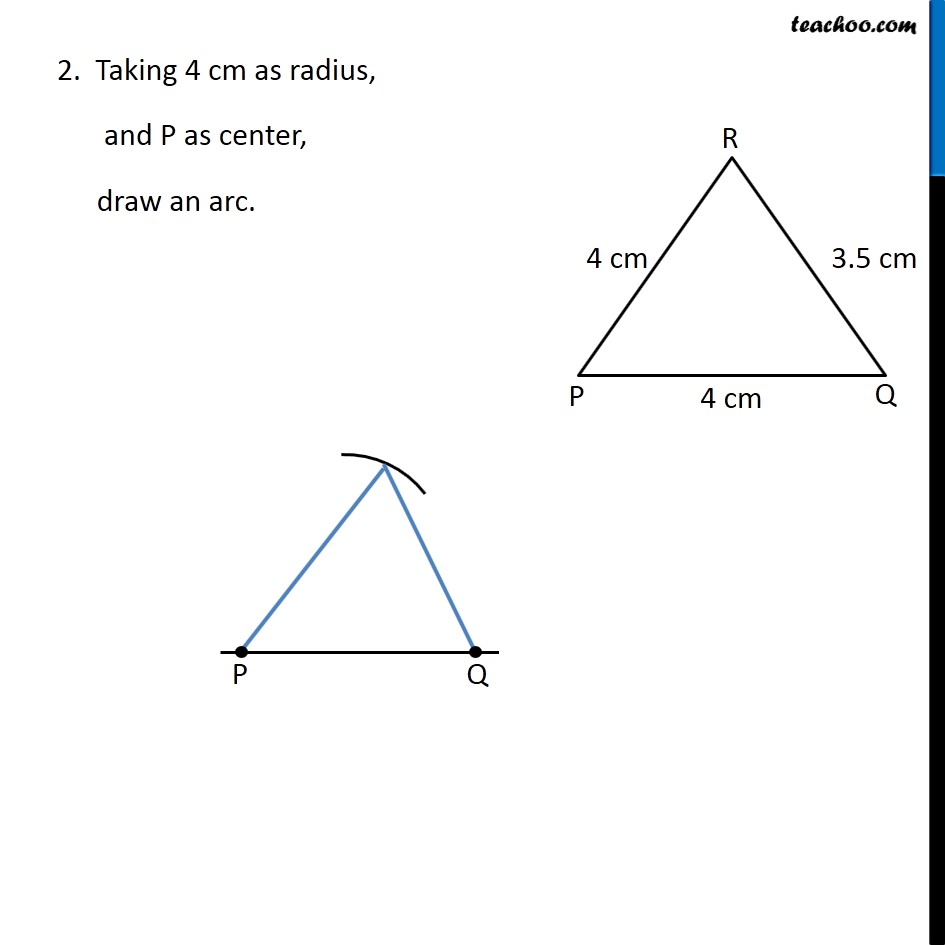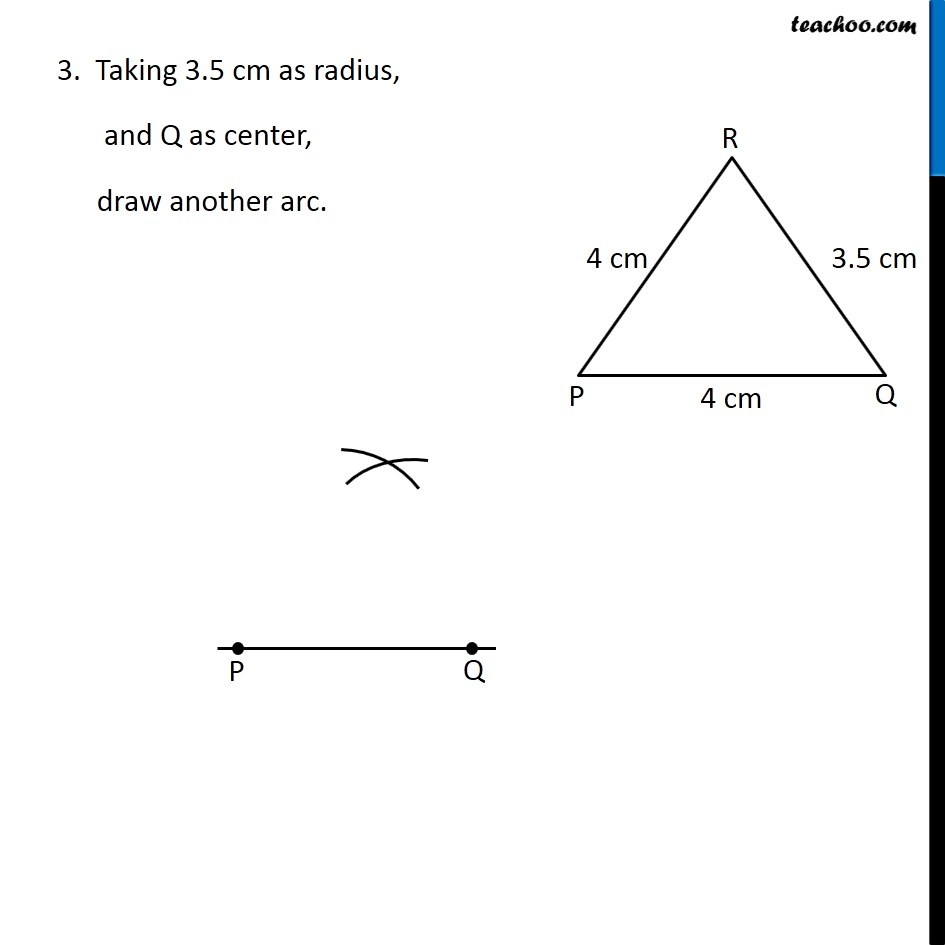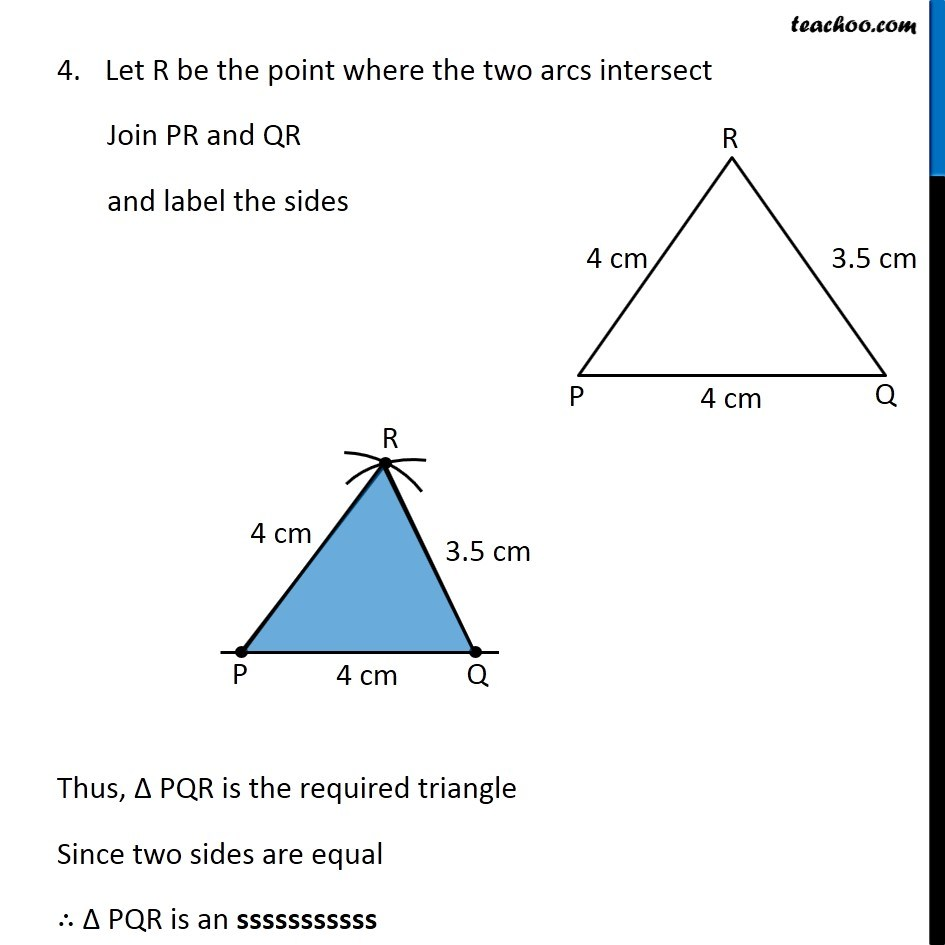Learn in your speed, with individual attention - Teachoo Maths 1-on-1 Class

### Transcript

Question 3 Draw ∆PQR with PQ = 4 cm, QR = 3.5 cm and PR = 4 cm. What type of triangle is this? First we draw a rough sketch We follow these steps Steps of construction 1. Draw a line segment PQ of length 4 cm 2. Taking 4 cm as radius, and P as center, draw an arc. 3. Taking 3.5 cm as radius, and Q as center, draw another arc. 4.Let R be the point where the two arcs intersect Join PR and QR and label the sides Let R be the point where the two arcs intersect Join PR and QR and label the sides Thus, Δ PQR is the required triangle Since two sides are equal ∴ Δ PQR is an sssssssssss Let R be the point where the two arcs intersect Join PR and QR and label the sides Thus, Δ PQR is the required triangle Since two sides are equal ∴ Δ PQR is an sssssssssss